# Calculate Optimum lamp height for maximum illuminance

A lamp is on point B (eg dining table) be suspended so that the illuminance at point A (eg table edge) is at a maximum. Thus, the optimum lamp position with maximum brightness would be found at the table edge.

At what height h the lamp should be suspended over the table?

### sketch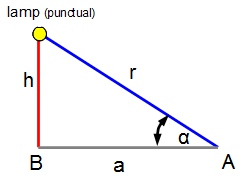The illuminance is proportional to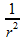and the projected area.
Thus: illuminance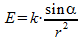. k is a proportionality factor.

Furthermore, the following applies: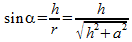.
Illuminance: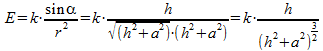.

Forming the 1st derivative to find the maximum: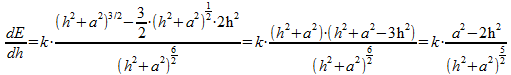.

Thus, the first derivative is zero, the counter must be zero.

## It follows for the extremes:.
(Note: That Extrema is a maximum because of the extremes on the h-axis pass through a sign change of + ("uphill") to - ("downhill") takes place alternatively use 2nd derivative..)

## Hence the optimum lamp height: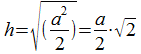.

## Example "lamp on a table" has h = 70 cm above the table when the table has a diameter of 2 m (a = 100 cm).

Have fun hanging lamp.